# RS Aggarwal Solutions for Class 6 Chapter 11 Line Segment, Ray and Line Exercise 11A

Exercise 11 A of RS Aggarwal Solutions provides the students with a clear idea about the basic concepts like planes, points and line segments. The solutions are depicted with pictorial representation to make the concepts easy for the students. The students can self analyse the areas which requires much practice from exam point of view. Here the students can get free PDF of RS Aggarwal Solutions for Class 6 Chapter 11 Line Segment, Ray and Line Exercise 11A.

## Download PDF of RS Aggarwal Solutions for Class 6 Chapter 11 Line Segment, Ray and Line Exercise 11A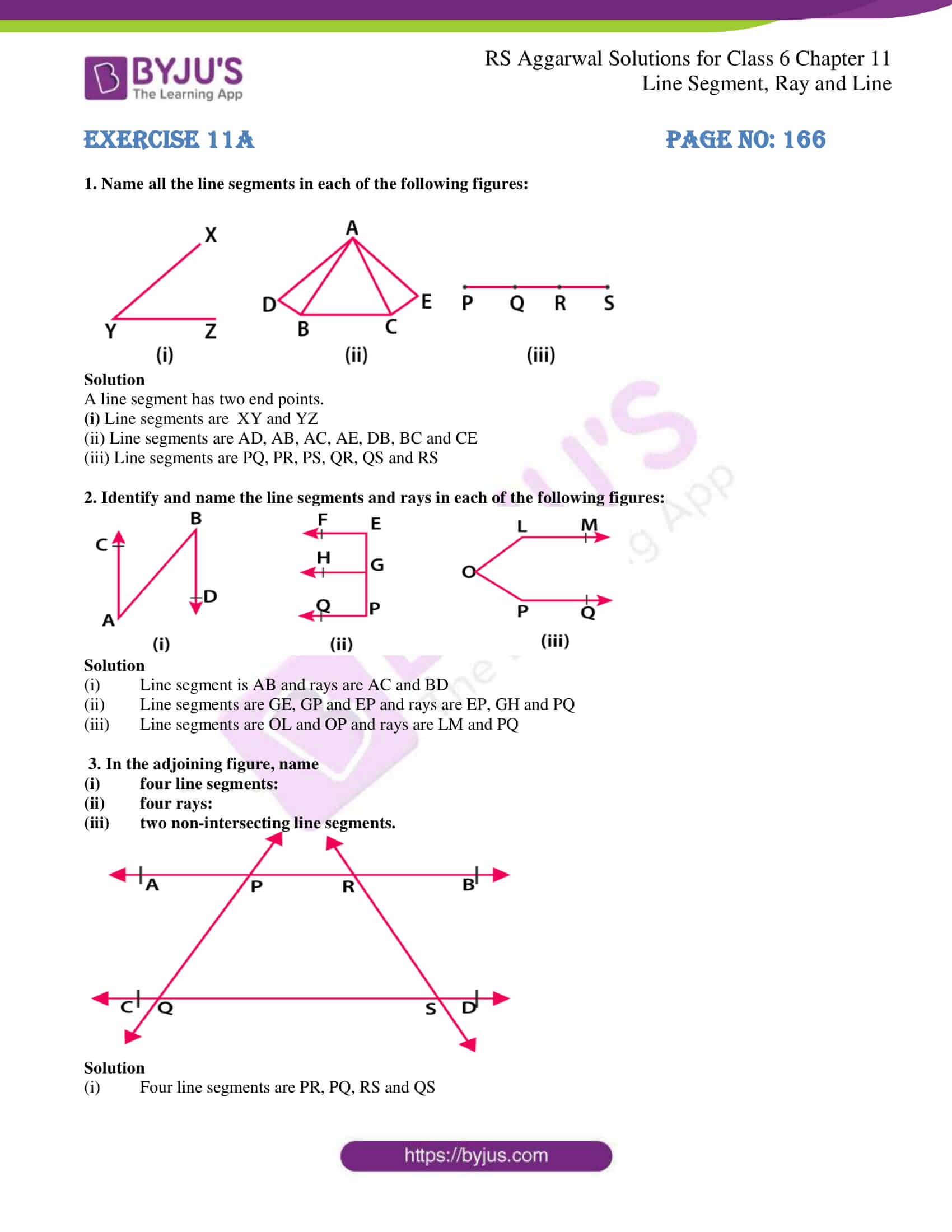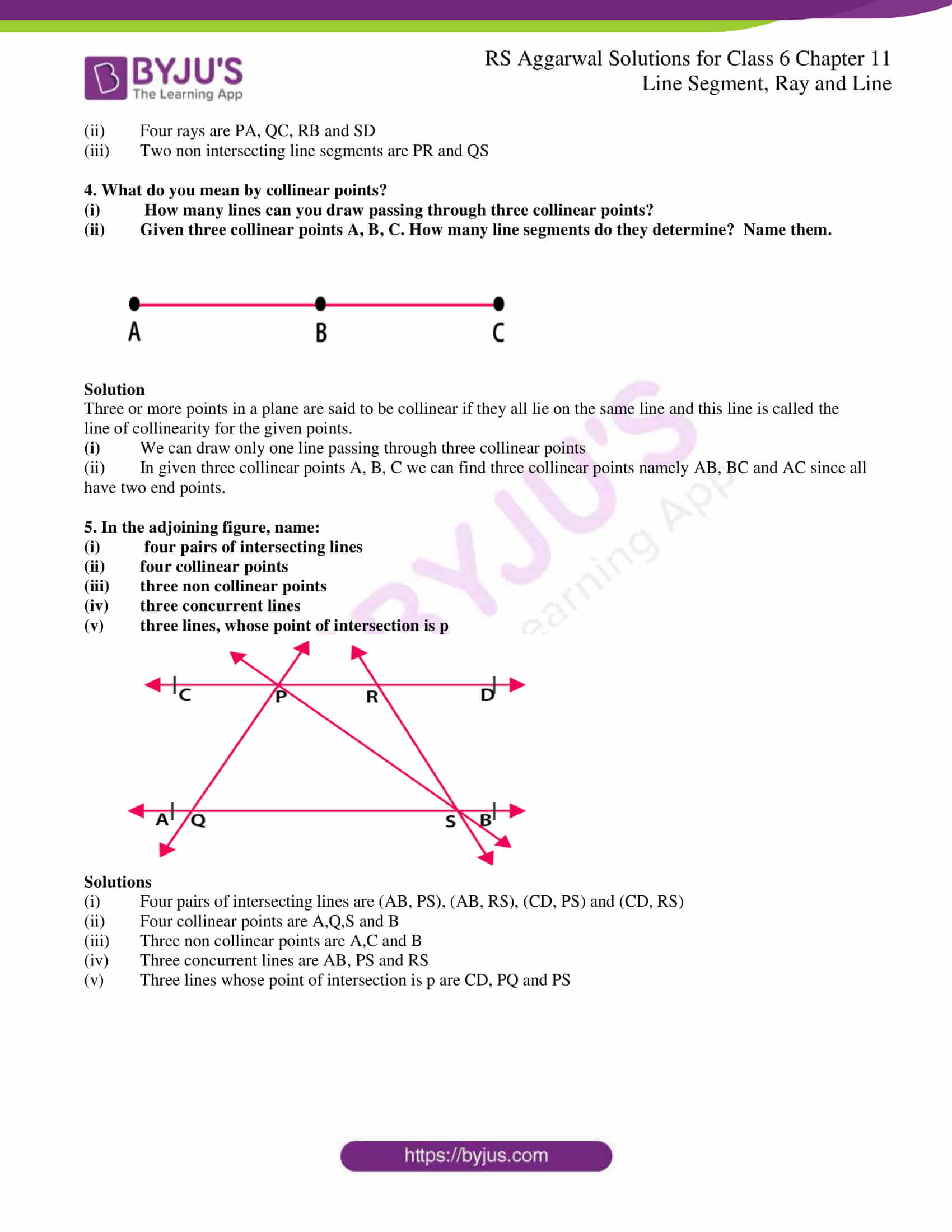### Access answers to Maths RS Aggarwal Solutions for Class 6 Chapter 11 Line Segment, Ray and Line Exercise 11A

1. Name all the line segments in each of the following figures: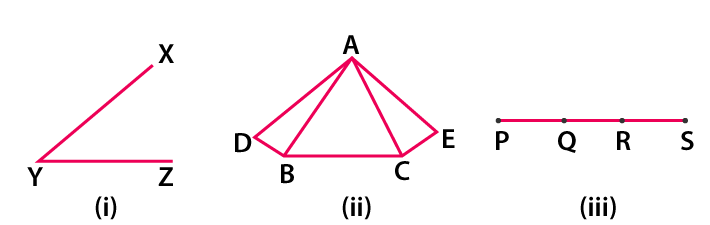Solution

A line segment has two end points.

(i) Line segments are XY and YZ

(ii) Line segments are AD, AB, AC, AE, DB, BC and CE

(iii) Line segments are PQ, PR, PS, QR, QS and RS

2. Identify and name the line segments and rays in each of the following figures: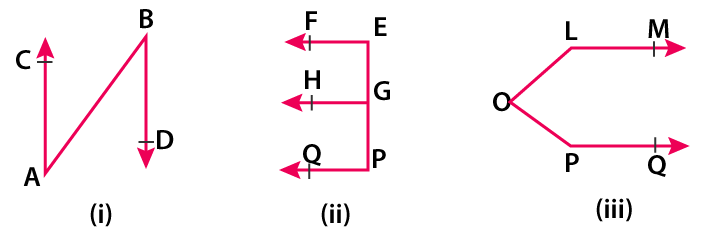Solution

(i) Line segment is AB and rays are AC and BD

(ii) Line segments are GE, GP and EP and rays are EP, GH and PQ

(iii) Line segments are OL and OP and rays are LM and PQ

3. In the adjoining figure, name

(i) four line segments:

(ii) four rays:

(iii) two non-intersecting line segments.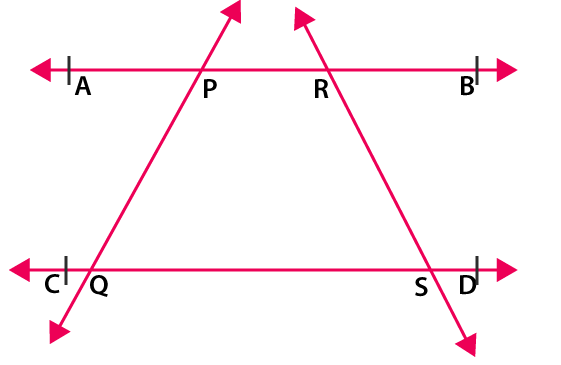Solution

(i) Four line segments are PR, PQ, RS and QS

(ii) Four rays are PA, QC, RB and SD

(iii) Two non intersecting line segments are PR and QS

4. What do you mean by collinear points?

(i) How many lines can you draw passing through three collinear points?

(ii) Given three collinear points A, B, C. How many line segments do they determine? Name them.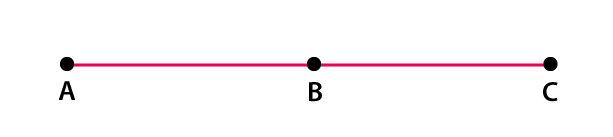Solution

Three or more points in a plane are said to be collinear if they all lie on the same line and this line is called the line of collinearity for the given points.

(i) We can draw only one line passing through three collinear points

(ii) In given three collinear points A, B, C we can find three collinear points namely AB, BC and AC since all have two end points.

5. In the adjoining figure, name:

(i) four pairs of intersecting lines

(ii) four collinear points

(iii) three non collinear points

(iv) three concurrent lines

(v) three lines, whose point of intersection is p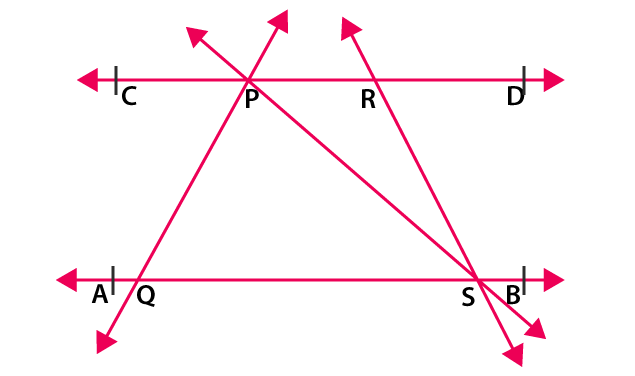Solutions

(i) Four pairs of intersecting lines are (AB, PS), (AB, RS), (CD, PS) and (CD, RS)

(ii) Four collinear points are A,Q,S and B

(iii) Three non collinear points are A,C and B

(iv) Three concurrent lines are AB, PS and RS

(v) Three lines whose point of intersection is p are CD, PQ and PS

### Access other exercises of RS Aggarwal Solutions for Class 6 Chapter 11 Line Segment, Ray and Line

Exercise 11B Solutions